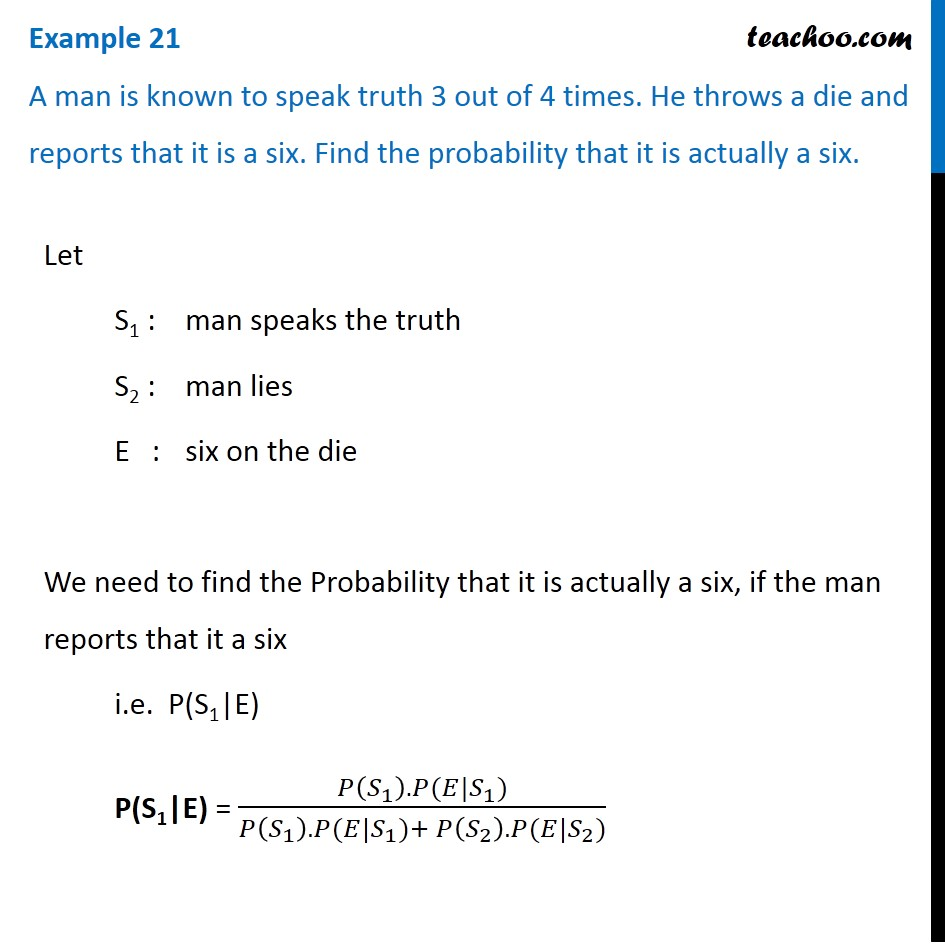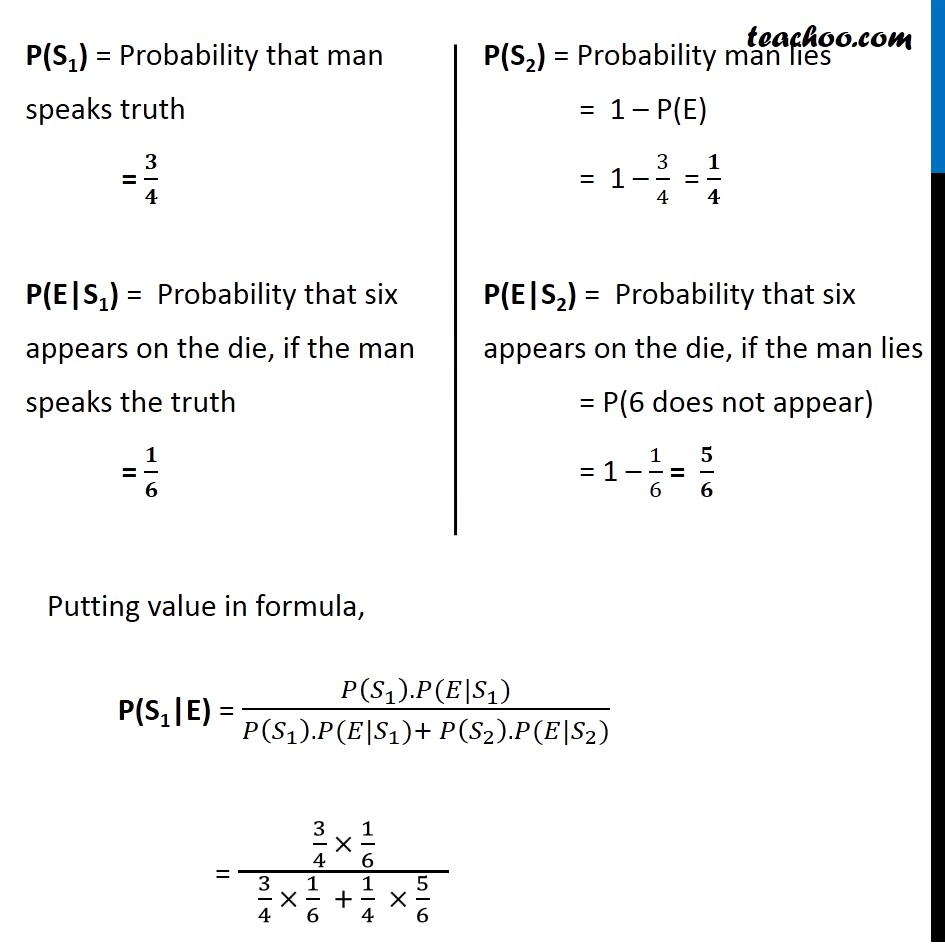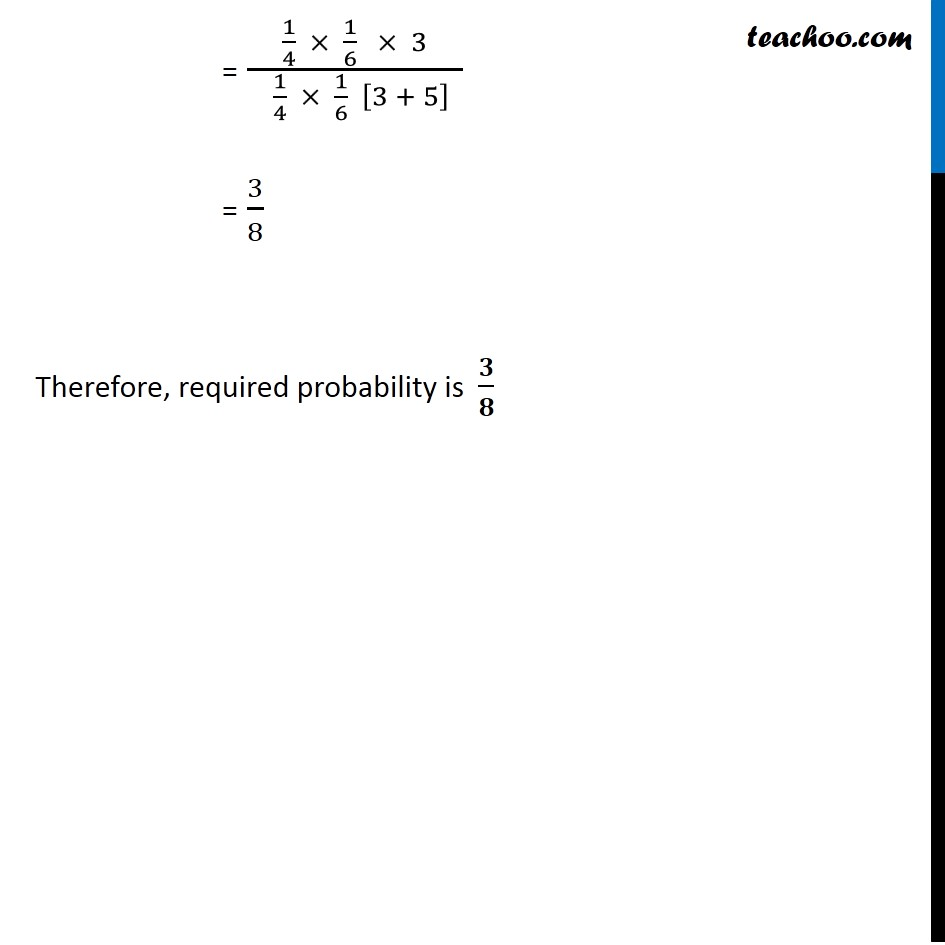Examples

Chapter 13 Class 12 Probability
Serial order wiseLearn in your speed, with individual attention - Teachoo Maths 1-on-1 Class

### Transcript

Example 21 A man is known to speak truth 3 out of 4 times. He throws a die and reports that it is a six. Find the probability that it is actually a six.Let S1 : man speaks the truth S2 : man lies E : six on the die We need to find the Probability that it is actually a six, if the man reports that it a six i.e. P(S1|E) P(S1|E) = (𝑃(𝑆_1 ).𝑃(𝐸|𝑆_1))/(𝑃(𝑆_1 ).𝑃(𝐸|𝑆_1)+𝑃(𝑆_2 ).𝑃(𝐸|𝑆_2)) P(S1) = Probability that man speaks truth = 𝟑/𝟒 P(E|S1) = Probability that six appears on the die, if the man speaks the truth = 𝟏/𝟔 P(S2) = Probability man lies = 1 – P(E) = 1 – 3/4 = 𝟏/𝟒 P(E|S2) = Probability that six appears on the die, if the man lies = P(6 does not appear) = 1 – 1/6 = 𝟓/𝟔 Putting value in formula, P(S1|E) = (𝑃(𝑆_1 ).𝑃(𝐸|𝑆_1))/(𝑃(𝑆_1 ).𝑃(𝐸|𝑆_1)+𝑃(𝑆_2 ).𝑃(𝐸|𝑆_2)) = (3/4 × 1/6)/( 3/4 × 1/6 + 1/4 × 5/6 ) = (1/4 × 1/6 × 3)/( 1/4 × 1/6 [3 + 5] ) = 3/8 Therefore, required probability is 𝟑/𝟖